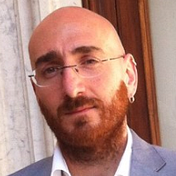# Math in Unity : create a “graphic grid” with Cartesian Plane and Line Renderer (Part I)

After creating our Cartesian plane, we will create a sort of “grid” based on the previous code.

First of all, inside CartesianPlane script, let’s create a double variable for the X and Y and serialize them.

The code inside Start () will now look like this

Now, to create a grid, we will use a for loop.
And we will create the grid by creating “quadrants”.
For example like this :

xMax is 160 or -160
yMax is 100 or -100

The logic is: the first loop follows the line of Vector X, between -160 and 160, and each “n value” (in this case 20, but soon we will serialize it to do other tests) draws Vector Y.
Same thing for the second loop, but I follow the Y line and draw the X Vector.

Since this is purely an exercise to understand how vectors work, the “size” of our quadrants will be the same, so our grid will always be made up of squares.
Of course, feel free to experiment.

Now, let’s serialize our size

And let’s use it within our loops

Test it

This works without problems because both 160 and 100 are divisible by 20.
But if for example you changed the size to 15, the result would be this

As you can see, of course it works, but we have an annoying offset that moves the grid from the point of origin.
You will also have noticed that when the grid is slightly displaced, the two lines of the Cartesian plane are again visible.

To solve the second problem, a simple solution is to add a float as a parameter of the DrawCartesianPlane () method.

in this way we could set the dimension of the THICKNESS of the line

We then create a float width as a parameter, and pass it into the lineRenderer as startWidth and endWidth.

Now in this part we will have some easily manageable errors by adding the missing parameter

after the changes

By setting the lines of our Cartesian plane to 1f and the grid lines to 0.5f, the result, with size = 20, will be this

In the next article we solve the second problem with a formula to set the offSet.

--

--Always in love with programming, even if late (I'm already 39 years old) I decided to follow my dream! We will see how far my passion will take me!